## Climb Performance

Once the aircraft has left the ground and a constant speed climb established, then climb performance can be simply calculated using a balance of the forces acting on the climbing vehicle.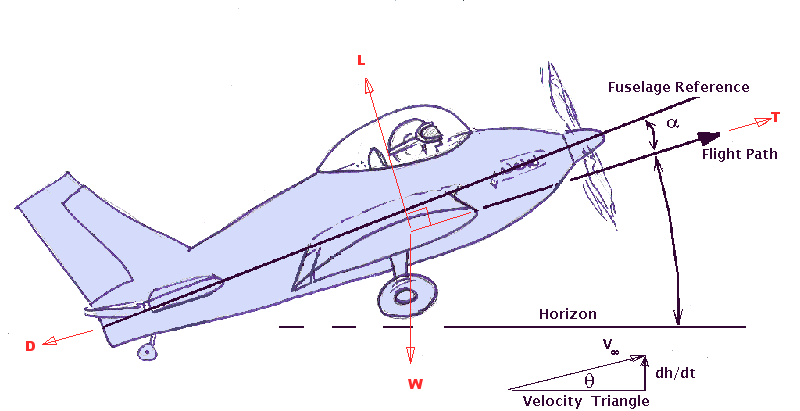In diagram the vehicle is assumed to be climbing at a constant angle (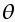) and
at a constant rate (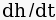)

The balance of forces in the direction perpendicular to aircraft flight (z-wind-axis) will give :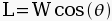The balance of forces in the direction parallel to the aircraft flight path (x-wind-axis) will give :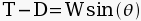Normal aircraft climb at relatively small angles so making the small angle assumption that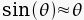, allows the following prediction of climb angle,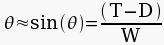Then by observing the relationship between climb angle, climb rate and flight velocity,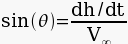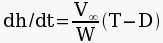The use of these equations in a numerical scheme is relatively straight forward. At a given aircraft weight, altitude and flight speed it should be possible to estimate the current Thrust (T) being produced and the current Drag (D) (see previous section on calculation of thrust and drag).

The difference between thrust and drag at the specified flight condition can hence be used to calculate climb angle and climb rate for the aircraft. Clearly if T=D then the aircraft is not climbing or descending but is in level flight. Also a obvious consequence is that if T<D then the aircraft must be descending.

These combination of terms :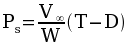is quite important in the evaluation of aircraft performance.

It is given the name Specific Excess Power ( Ps ). The fact that the terms have velocity * force units involved makes this a term in power; excess power, because of the assumption that T * V, power available from engines, is greater than D * V, power required to overcome energy loss due to motion.  The Ps term is denoted as Specific since the power terms are divided by the weight of the vehicle so that it is not being used in absolute terms but relative to the weight of the vehicle.

For this steady flight analysis Specific Excess Power will create a climb rate but for dynamic motion of the aircraft it can be used to climb, accelerate or turn. This will be covered in later chapters.

Investigation of the Specific Excess Power term over a range of flight speeds in the initial climb at sea level conditions can be used to find important results. Using the above simple equations in a spreadsheet for example can be used to predict the flight speed to use that will maximise the climb rate or the climb angle (Note: These two optima may not occur at the same flight speed).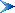Table of Contents

© AMME, University of Sydney, 1998-2006.